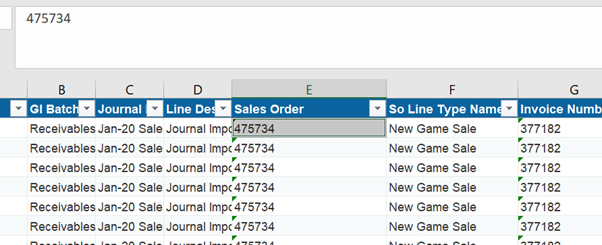# Unable to add SUMIF formula for multiple columns are in multiple sheets

Hi,

I’m trying to apply SUMIF formula for multiple columns that are in different excel sheets.

Below is the SUMIF formula I’m trying to apply.

=SUMIF(Revenue!E:E,Summary!A2,Revenue!AE:AE)

Here “Revenue” is a different sheet where we are looking entire Column’s-“E” & “AE”.
“Summary” is a different sheet where we are using the Column Cell - “A2” and write the SUMIF value in Summary Sheet - column Index =4.

Can you please suggest any idea how I can read 2 excels and apply SUMIF formula for both the sheets.
Below are the sample excel sheet screenshots.Are you able to apply the formula manually in the excel?

Hi,

Yes…I’m able to apply the formula(=SUMIF(Revenue!E:E,Summary!A2,Revenue!AE:AE)) manually and getting the data. Below is Output value which I’m writing in the column -E(Summary Sheet).

use write cell acitivity and check if you are able to write formula to one cell and see if it works fine and confirm

Create Macro in excel to perform SUMIF and use Execute Macro activity in UiPath to generate the results through Macro.

Hi,

I need to download the multiple excels , filter with specified data and then make a consolidated sheet where applying VlookUp and SUMIF for many column’s of data. and output data has to be appended in summary sheet based on each run.

Soo i think Creating a macro in excel and applying formula would make it complicate. Can you please suggest any other idea?.

Hi,

I have an idea to write the formula in Write Cell. But here i’m referring 2 column’s from 1 sheet and other 2 column’s from another sheet. and that’s where i’m stuck how to write the condition for 2 sheets.

I’m storing the 2 sheets of data in 2 Data tables.but not getting any idea to write the formula - =SUMIF(Revenue!E:E,Summary!A2,Revenue!AE:AE)

Need not worry about that.If manually a formula is working, writing the same formula using write cell would work same as manual step

Tried. But It’s not giving any output.Just created a sample project.
Try this and follow.
here → UiPath/ApplyFormulaToColumn.xaml at master · arunvelaayudhan/UiPath · GitHub

What is the problem here? The formula is becoming like text or you need help to build the formula dynamically?

Attached are ther 2 Excel sheets and the WF.

the Column Name - “Sales Order” is common in both summary sheet and revenue sheet.

So i’m reading the both data tables and fetching the column"Sales Order" data - and assigning to a data row. Now for item in DataRow…I’m applying the below formula to sum the Amount(Column -AE) of each sales order which is in the range(E Column in Revenue sheet).
So instead of giving the sum value, it’s returing 0.

Can you please check the Workflow and the excel sheet and help me?

Sample WF.zip (154.1 KB)

1 Like

The formula must be applied to the excel and not the datarow/datatable… but if you need to use the result of the formula being applied in a process, then i think we should go with a different approach…

1 Like

Then can you please suggest me the other approach as I don’t have any idea how to develop.

1 Like

I still not sure if you used Write Cell activity to write that formula to the cell you want or not…

I wrote the formula that we actually enter manually in write cell and tired running… But it didn’t give any output…
That’s why assigned the conditions and tried to show the output in message box before writing it in excel. Can you please refer to the excel

I used write cell as you mentione. But it’s not it’s writing anything. Column is blank.

This topic was automatically closed 3 days after the last reply. New replies are no longer allowed.